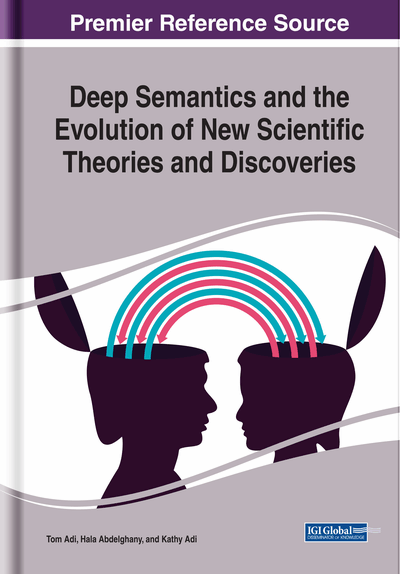# Observations From Word Roots About Mathematics

DOI: 10.4018/978-1-5225-8079-9.ch007

## Abstract

In this chapter, the authors discuss the meanings of the sounds of Arabic word roots for numbers.
Chapter Preview
Top

## The Number Four: Addition, Duplication, And Multiplication

Divine inspirations about deep semantics (meanings of sounds) started in 1985. However, the meanings of the sounds that give us the Arabic word roots for numbers remained a mystery until 2017 when some inspirations about the number four were recorded (Adi, 2017). The last two word roots discussed in Chapter 6, RBW (unjustified profit) and RBH (profit) have structures that are similar to the word root for the number four: RBO.

All three word roots deal with numbers and increased amounts. However, the meanings of the sounds of the word root for the number four (RBO) introduce us in a clear way to the concepts of addition, duplication, and multiplication. Let’s take a close look.

Figure 1 illustrates the word root “ربع RBO” (the number four). The contents of this figure and its discussion below consist of speculations that are based on short-lived Divine inspirations that we received about this word root and recorded in the past.

In the word root RBO, both R and B represent the Divine general process type “manifest + assign” which is lighter than the general process represented by O (see Figure 1 in Chapter 3 for the weights of Divine general process types).

Because R and B are adjacent to each other, the type of Divine process control flow is Two Nested Control Zones that control a third general process (see the overview of the types of Divine process control flow in Figure 21, and an illustration of this type of Divine process control flow for a similarly structured word root in Figure 15, both figures are in Chapter 3).

The general process represented by B is a Joiner which controls the general process represented by O. This control operation is nested inside the control zone of R which represents a Backward Controller.

The sound R represents the Divine general process “closed-selfmanifest + assign.”

Although the shape of the control zone of R reflects its process mode “closed-self” as the concept “back” which we find under the header “Closed Self” in Figure 3 in Chapter 4, the meaning it gives us for this word root is repeat which we also find under “Closed Self” in Figure 3 in Chapter 4 (see the right side of Line 2 in Figure 1).

The compound Divine general process type represented by the sound R “manifest + assign” means “allocate amount,” a group of meanings from the section “Manifest + Assign” in Figure 2 in Chapter 4. The meaning chosen is “calculate” for this word root (see the right side of line 2 in Figure 1).

Based on the above, the Backward Controller represented by the sound R means “repeat calculate” or “duplicate” (see the right side of line 2 in Figure 1). This introduces the arithmetic concept of “duplication” which can be generalized to give us the concept of “multiplication.”

The Joiner represented by B is the Divine general process “closed-groupmanifest + assign.”

## Complete Chapter List

Search this Book:
Reset